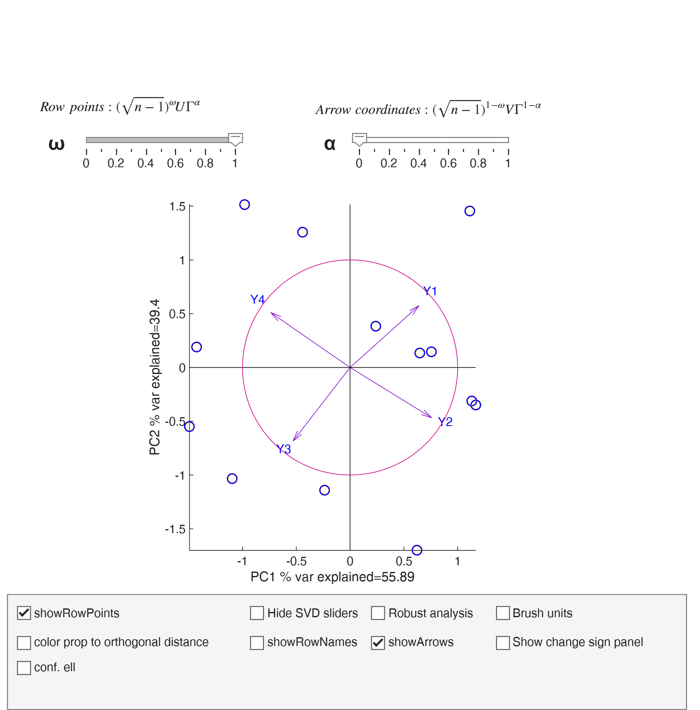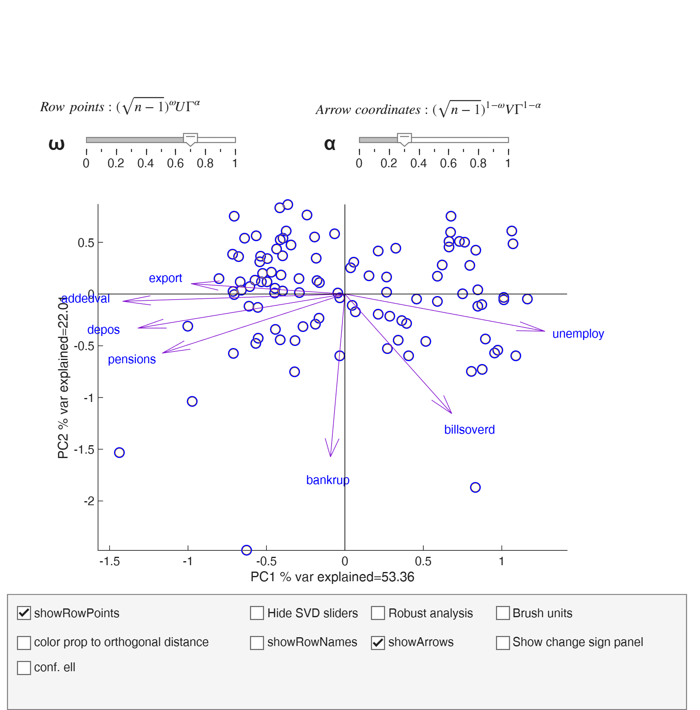# biplotFS

biplotFS calls biplotAPP.mlapp to show a dynamic biplot

## Syntax

• biplotFS(Y)example
• biplotFS(Y,Name,Value)example

## Description

The svd of $Z=zscore(Y)$ $Z= U \Gamma^* V^T$ and its best rank two approximation $Z \approx U_{(2)} \Gamma_{(2)}^* V_{(2)}^T$

where $U_{(2)}$ is $n \times 2$ matrix (first two columns of $U$) and $V_{(2)}$ is $p \times 2$ (first two columns of matrix $V$, $\Gamma_{(2)}^*$ is a $2 \times 2$ diagonal matrix which contains the frist two largest singular values of matrix $Z$ (square root of the eigenvalues of matrix $Z^TZ=(n-1)R$) where $R$ is the correlation matrix.

We can also write

$\frac{Z}{\sqrt{n-1}} \approx U_{(2)}\frac{\Gamma_{(2)}^*}{\sqrt{n-1}} V_{(2)}^T$ $Z \approx \sqrt{n-1} U_{(2)}\frac{\Gamma_{(2)}^*}{\sqrt{n-1}} V_{(2)}^T$ $Z \approx \sqrt{n-1} U_{(2)} \Gamma_{(2)} V_{(2)}^T$

where $\Gamma_{(2)} = \Gamma_{(2)}^*/ \sqrt{n-1}$.

In this way $\Gamma_{(2)}$ contains the singular values (square root of the eigenvalues) of matrix $Z^TZ/(n-1)=R$ (correlation matrix).

The last equation can be written as function of two parameters $\alpha \in [0 1]$ and $\omega \in [0 1]$

$Z \approx \left[ (\sqrt{n-1})^\omega U_{(2)} \Gamma_{(2)}^\alpha \right] [ \Gamma_{(2)}^{1-\alpha} V_{(2)}^T (\sqrt{n-1})^{1-\omega} ]$ In the dynamic biplot the $n$ row points are represented by $n \times 2$ matrix: $\left[ (\sqrt{n-1})^\omega U_{(2)} \Gamma_{(2)}^\alpha \right]$ and the $p$ column points (arrows) through $2 \times p$ matrix $[ \Gamma_{(2)}^{1-\alpha} V_{(2)}^T (\sqrt{n-1})^{1-\omega} ]$ Note that if $\omega=1$ and $\alpha=0$ row points are $\sqrt{n-1} U_{(2)}= Z V_{(2)} \Gamma_{(2)}^{-1}$

that is row points are the standardized principal components scores.

$cov (\sqrt{n-1} U_{(2)}) = I_2= \left( \begin{array}{cc} 1 & 0 \\ 0 & 1 \\ \end{array} \right)$ Column points are $\Gamma_{(2)} V_{(2)}^T$

that is the arrows are associated with the correlations between the variables and the first two principal components The length of the arrow is exactly equal to the communality of the asoociated variable.

In this case the unit circle is also shown on the screen and option axis equal is set.

On the other hand, if $\omega=1$ and $\alpha=1$ the $n$ rows of $Z$ (of dimension $n \times p$) can be represented using $n \times 2$ matrix:

$\sqrt{n-1} U_{(2)} \Gamma_{(2)} = Z V_{(2)}$ In this case the row points are the (non normalized) scores, that is $cov(\sqrt{n-1} U_{(2)} \Gamma_{(2)}) =cov( Z V_{(2)})= \left( \begin{array}{cc} \lambda_1 & 0 \\ 0 & \lambda_2 \\ \end{array} \right)$ With this parametrization the column points (arrows) are nothing but the coordinates of the first two eigenvectors $V_{(2)}^T$

Also in this case the unit circle is given and option axis equal is set.

In general if $\omega$ decreases, the length of the arrows increases and the coordinates of row points are squeezed towards the origin In the app it is also possible to color row points depending on the orthogonal distance ($OD_i$) of each observation to the PCA subspace.

For example, if the subspace is defined by the first two principal components, $OD_i$ is computed as:

$OD_i=|| z_i- V_{(2)} V_{(2)}' z_i ||$ If optional input argument bsb or bdp is specified it is possible to have in the app two tabs which enable the user to select the breakdown point of the analysis of the subset size to use in the svd. The units which are declared as outliers or the units outside the subset are shown in the plot with filled circles.

 biplotFS(Y) use of biplotFS on the ingredients dataset.

 biplotFS(Y, Name, Value) use of biplotFS on the dataset referred to Italian cities.

## Examples

expand all

###use of biplotFS on the ingredients dataset.

load hald
% Operate on the correlation matrix (default).
% use standardized principal components (for row points) and
% correlation between variables and principal components (for column
% points, arrows)
close all
biplotFS(ingredients,'omega',1,'alpha',0);
When \omega=1 and \alpha=0
Row points represent standardized principal components sqrt(n-1)*U=ZV*\Gamma^-1
Arrows represent correlations between variables and principal components \Gamma*V
The length of the arrows is the communality of the variable
In this case the unit circle is also shown###use of biplotFS on the dataset referred to Italian cities.

load citiesItaly
biplotFS(citiesItaly)## Input Arguments

### Y — Input data. 2D array or table.

n x v data matrix; n observations and v variables. Rows of Y represent observations, and columns represent variables.

Missing values (NaN's) and infinite values (Inf's) are allowed, since observations (rows) with missing or infinite values will automatically be excluded from the computations.

Data Types: single|double

### Name-Value Pair Arguments

Specify optional comma-separated pairs of Name,Value arguments. Name is the argument name and Value is the corresponding value. Name must appear inside single quotes (' '). You can specify several name and value pair arguments in any order as  Name1,Value1,...,NameN,ValueN.

Example:  'bsb',[2 10:90 93] , 'bdp',0.4 , 'standardize',false , 'alpha',0.6 , 'omega',1 , 'standardize',false , 'showRowNames',false , 'showArrows',false 

### bsb —units forming subset on which to perform PCA.vector.

Vector containing the list of the untis to use to compute the svd. The other units are projected in the space of the first two PC. bsb can be either a numeric vector of length m (m<=n) containin the list of the units (e.g. 1:50) or a logical vector of length n containing the true for the units which have to be used in the calculation of svd. For example bsb=true(n,1), bsb(13)=false; excludes from the svd unit number 13.

Note that if bsb is supplied bdp must be empty.

Example:  'bsb',[2 10:90 93] 

Data Types: double or logical

### bdp —breakdown point.scalar.

It measures the fraction of outliers the algorithm should resist. In this case any value greater than 0 but smaller or equal than 0.5 will do fine. Note that if bdp is supplied bsb must be empty.

Example:  'bdp',0.4 

Data Types: double

### standardize —standardize data.boolean.

Boolean which specifies whether to standardize the variables, that is we operate on the correlation matrix (default) or simply remove column means (in this last case we operate on the covariance matrix).

Example:  'standardize',false 

Data Types: boolean

### alpha —svd parameter.scalar.

Scalar in the interval [0 1] (see section additional details for more help). This parameter can be controllad by the corresponding sliding bar when the app is shown.

Example:  'alpha',0.6 

Data Types: double

### omega —svd parameter.scalar.

Scalar in the interval [0 1] (see section additional details for more help). This parameter can be controllad by the corresponding sliding bar when the app is shown.

Example:  'omega',1 

Data Types: double

### showRowPoints —hide or show row point.boolean.

If showRowPoints is true row points are shown in the biplot (default) else there are hidden.

Example:  'standardize',false 

Data Types: boolean

### showRowNames —hide or show labels of row points.boolean.

If showRowNames is true labels of row names are shown in the biplot else (default) there are hidden.

Example:  'showRowNames',false 

Data Types: boolean

### showArrows —hide or show arrows.boolean.

If showArrows is true arrows (associated labels) labels are shown in the biplot (default) else there are hidden.

Example:  'showArrows',false 

Data Types: boolean

## Output Arguments

when the biplotAPP is closed in the base workspace a new variable called bsbfinalFromAPP is created which contains a logical vector of length n containing true for the units which have been used in the svd.